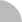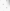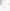# 臺灣博碩士論文加值系統

(34.204.181.91) 您好！臺灣時間：2023/10/01 13:27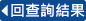:::

### 詳目顯示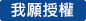: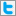Twitter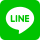•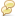被引用:0
•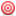點閱:100
•評分: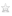•下載:0
•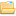書目收藏:0
 本論文包含下列三個主要定理，第一個定理處理Shih-Ho的猜想。 定理1。令F : {0, 1}^n-->{0, 1}^n.假設對所有x屬於{0, 1}^n，恒有 ρ(F''(x))=0,則F有唯一的固定點。 定理2。令F:{0, 1}^n-->{0, 1}^n.假設對所有x屬於{o, 1}^n，恒有 ρ(f''(x))=0，則F有唯一的固定點ξ。此外，假設對所有 x屬於V_{n-2}(ξ)，則ξ是強吸子，這V_(n-2)(ξ)是最佳的。 定理3。令F:{0, 1}^n-->{0, 1}^n。假設對所有x屬於{0, 1}^n。恒有 ρ(F''(x))=0，而且對所有x屬於{0, 1}^n恒有F(V_1(x))包含於 V_1(F(x))。則對每一個x屬於{0, 1}^n存在一唯一點 _ α屬於x[{1,...,n-1}|n]及唯一點β屬於x[{1,...,n-1}|n]使得對所有j=1,...n-1，f_j(α)=α_j及f_j(β)=β_j更進一步。如果ρ_H(α,β)=n，則存在兩個正整數p,q小於等於n，使得對所有x屬於{0, 1}^n恒有F^p(x)=α及G^q(x)=α或者對所有x屬於{0, 1}^n恒有F^p(x)=α及G^q(x)=α。對於Shih-Ho的猜想之動機是來自於代數幾何的Jacobian猜想。在1939年由數學家O.H.Keller所提出的，在1995年，這三位數學家Cima, Gasull及Manosas証明這傑出的Jacobian猜想等價於這個敘述： 令F:C^n-->C^n是一個多項式映射(-i.e., 每一個 f_i 是一個多項式)而且對所有x屬於C^n恒有ρ(F''(x))<1，則F有唯一的固定點。本論文主要有三個層次。論文的第一個層次是去証明Shih-Ho的猜想。論文的第二個層次是去決定這最佳的Hamming球圈使得固定點在超立方體 {0, 1}^n是強吸子。論文的最三個層次是對Shih在Gauss-Seidel算子G之動態行為的猜想做部份的解答。本論文的動機主要是去研究自動機網路所激發的。至於這些書籍可參考我們的參考文獻。
 This thesis contains the following three main theorems, the first theorem settles the Shih-Ho''s conjecture. Theorem. Let F : {0, 1}^n --> {0, 1}^n. If ρ(F''(x)) = 0 for all x in {0, 1}^n, then F has a unique fixed point. Theorem. Let F : {0, 1}^n --> {0, 1}^n. If ρ(F''(x)) = 0 for all x in {0, 1}^n, then F has a unique fixed point ξ. If, in addition, F(V_1(x)) included in V_1(F(x)) for all x in V_{n-2}(ξ), then ξ is a strongly global attractor. The V_{n-2}(ξ) is optimal. Theorem. Let F : {0, 1}^n --> {0, 1}^n. Suppose ρ(F''(x)) =0 for all x in {0, 1}^n, and F(V_1(x)) included in V_1(F(x)) for all x in {0, 1}n. Then for each x in {0, 1}^n there exist a unique point α in x[{1, ..., n-1}|n] _ and a unique point β in x[{1, ..., n-1}\,|\,n] such that f_j(α) = α_j and f_j(β) = β_j for all j = 1, ..., n-1. Further, if ρ_H(α,β ) = n, then there exist two positive integers p, q less or equal to n such that F^p(x) =α and G^q(x) = α for all x in {0, 1}^n or F^p(x) =β and G^q(x) =β for all x in {0, 1}^n. Consider the set of all points in n-dimensional space with each coordinate equal to zero or one. These points may be thought of as the corners of a n-dimensional cube. We let these points correspond to processors, and we consider a communication link for every two points differing in a single coordinate. The resulting network is called a n-cube {0, 1}^n. The n-cube architecture has many attractive features (see Bertsekas and Tsitsiklis ). Theory of Automata Network was introduced by S. Ulam , W. McCulloch  and J. von Neumann (,). Automata Networks are discrete dynamical systems, in time and space. Roughly speaking , they are defined by a graph, where each vertex takes states in a finite set. Thus a discrete dynamical system is modelled by F : {0,1}^n --> {0, 1}^n. A basic theory of discrete dynamical system modelled by F : {0, 1}^n --> {0, 1}^n was given in Robert  and  (see also ,). A recent work by Shih and Ho  deepened the theory of Robert. Among the results of Shih and Ho, Shih and Ho made the following conjecture. Conjecture. Let F : {0, 1}^n --> {0, 1}^n. If ρ(F''(x)) = 0 for all x in { 0, 1}^n, then F has a unique fixed point. This conjecture was motivated by the outstanding Jacobian conjecture in Algebraic Geometry formulated by O. H. Keller in 1939 (see Bass, Connell and Wright ). In 1995, Cima , Gasull and Ma$\tilde{n}$osas proved that the Jacobian Conjecture is equivalent to the following : If F : C^n --> C^n is a polynomial mapping (i.e., each f_i is a polynomial) and ρ(F''(x)) < 1 for all x in C^n, then F has a unique fixed point. The purpose of this thesis has three folds. The first fold of this thesis is to prove Shih-Ho''s conjecture in Sect. 3, Theorem 3.1. The second fold of this thesis is to determine the optimal Hamming sphere such that the fixed point is strongly attractive in {0, 1}^n. Our answer of the optimal Hamming sphere is V_{n-2}(ξ). The main result is given in Theorem 4.1. The third fold of this thesis is to give a partial answer of the conjecture raised by Shih concerning the dynamical behavior of the Gauss-Seidel operator for F : {0, 1}^n --> {0, 1}^n. The main result is given in Theorem 5.1.The content of this thesis is organized as follows. Section 2 is a development of the tools and the spectra of Boolean matrices, needed to prove the main theorem. Section 3, the main theorem of this thesis, is to prove Shih-Ho''s conjecture. Section 4 gives a study of dynamical behavior of hypercube mappings. Section 5 is a study of Gauss-Seidel iteration. It should be mentioned here that the motivation of this thesis is motivated by the study of the Associated Memory in Automata Network, see for example, Fogelman, Robert and Tchuente , Goles and Martinez  , Kohonen , and Robert  and .
 封面中文摘要Abstract謝誌目錄１．緒論２．序言３．主要定理４．超立方體映射之動態行為５．離散型之 Gauss-Seidel 疊代６．參考文獻７．附錄【英文論文及參考文獻】
 1.H. Bass, E. H. Connell and D. Wright, The Jacobian Conjecture : Reduction of Degree and Formal Expansionof The Inverse, Bulletin (new series) of The Americal Mathematical Society, 7, (1982), no2, 287-330.2.D. P. Bertsekas, J. N. Tsitsiklis, Paralled and Distributed Computations : NumericalMethods, Prentice Hall, 1989.3.R. A. Brualdi and H. J. Ryser, Combinatorial Matrix Theory, Cambridge University Press, Cambridge,1991.4.A. Cima, A. Gasull, and F. Manosas, The discrete Markus-Yamabe problem, Prepulblications Nn''m. 26/1995 Universitat Autonoma de Barcelona, 1995.5.F. Fogelman, Y. Robert, and M. Tchuente, Automata Networks in Computer Science, Manchester University Press, 1989.6.E. Goles and S. Martinez, Neural and Automata Networks, Dynamical Behavior and Applications,Kluwer Academic Pubishers, Dordrecht-Boston-London, 1991.7.Kim, K. H. Boolean Matrix theory and Applications, Lecture Notes in Pure and Applied Mathematics, New York, Marcel Dekker Inc. (1982).8.T. Kohonen, Self-Organization and Associative Memory,Springer Series in Information Sciences, Springer-Verlag, Berlin-Heidelberg-New York, 1984.9.W. McCulloch, W. Pitts, A Logical Calculus of the Ideas Immanent in Nervous Activity, Bull. of Math. Biophysics, 5(1943) 115-133.10.J. P. LaSalle, The Stability of Dynamical Systems, Regional Conference Series in Applied Mathematics, 25, 1976.11.F. Robert, Iterations sur des ensembles finis et automates cellulaire contractants,Linear Algebra and Appl. 29 ( 1980 ), 393-412.12.F. Robert, Derivee discrite et convergence local dune iteration booleene,Linear Algebra and Appl. 52 ( 1983 ), 574-589.13.F. Robert, Discrete Iterations, A Metric Study, Springer Series in computational Mathematics,Springer-Verlag, Berlin-Heidelberg-New York, 1986.14.F. Robert, Les Systemes Dynamiques Discretes, Springer-Verlag Berlin Heidelberg New York 1994.15.M. H. Shih, J. L. Ho, Solution of the Boolean Markus-Yamabe Problem, Advances in Applied Math, 22 (1999) 60-102.16.S. Ulam, On Some Mathematical Problems Connected with Patterns of Growth of Figures, in Essays onCellular Automata, A. W. Burks(ed), Univ. of Illinois Press (1970) 219-243.17.J. Von Neumann, Theory of Self-Reproducing Automata, A. W. Burks(ed), Univ. of Illinois Press , 1966.18.J. Von Neumann, The General and Logical Theory of Automata, in Hixon Synposium Proc., 1948 in J. N. NeumannCollected Works, A. H. Toub(ed), Pergamon Press, V,(1963) 288-328.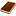國圖紙本論文推文當script無法執行時可按︰推文 網路書籤當script無法執行時可按︰網路書籤 推薦當script無法執行時可按︰推薦 評分當script無法執行時可按︰評分 引用網址當script無法執行時可按︰引用網址 轉寄當script無法執行時可按︰轉寄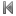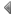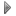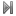top
 無相關論文

 無相關期刊

 1 生理訊號分析技術於刑案測謊之應用評估 2 雙影像量測IC接腳高度之可行性研究 3 公司新股上市時配售方式之選擇與影響 4 樊氏匹配定理之研究 5 嘉義縣市高齡學習參與者與非參與者在人際關係、生活滿意度之比較研究 6 電弧爐還原渣再生應用於高性能混凝土性質之研究 7 射出成型製程於導電性高分子複合材料EMI屏蔽效應之研究 8 室內設計居家工作者之特性研究 9 住居中的身體與生活光影--電影詩意空間情境的探索 10 圖書經銷商角色與功能之研究:農學社的個案研究 11 郵政支局經營效率評估之研究──以資料包絡分析法運用於基隆特等郵局所轄支局為例 12 機場鄰近地區風險量測與因應策略之研究 13 台灣半導體製造廠工業工程師工作領域之現況分析 14 彈性支承橋梁於移動載重作用下之動態互制分析 15 生態學及其在比較教育研究的意義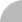簡易查詢 | 進階查詢 | 熱門排行 | 我的研究室